/

### Moments of a binomial loss distribution

Suppose a portfolio hasequally-sized exposures. Each one is independent and has a probability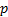of creating a unit loss (and a probability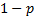of creating a zero loss), withthe same for each exposure, meaning that the portfolio loss,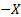, is distributed according to a binomial distribution, i.e.: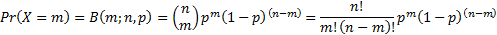The mean and the variance of the portfolio loss distribution can be found as follows. We note that: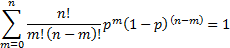The mean of the loss distribution is given by: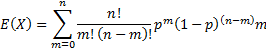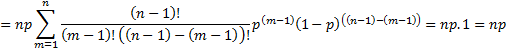Likewise: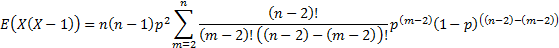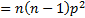The variance of the loss distribution is: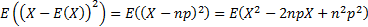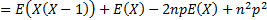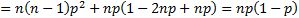Thus binomial distribution has mean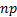and variance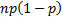.

As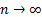, the Central Limit Theorem CLT implies that the binomial distribution tends to a normal distribution with the same mean and variance, i.e. to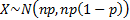where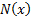is the cumulative normal distribution.